2018-06-09 17:15:06

qwq终于把splay调出来了。。。指针这东西有毒，所以换数组重打了一遍。。。

Splay的中文叫伸展树，并且具有二分查找树的性质，就是所有右子树都比根大，所有左子树都比根小。至于为什么叫伸展树，因为它最重要的操作叫做伸展。。

1.x（当前节点）的父亲节点为根节点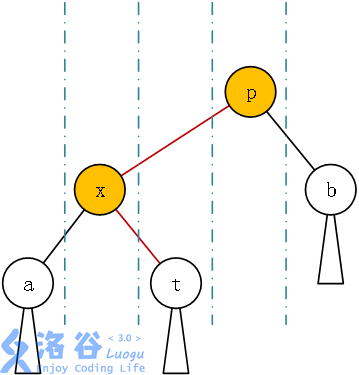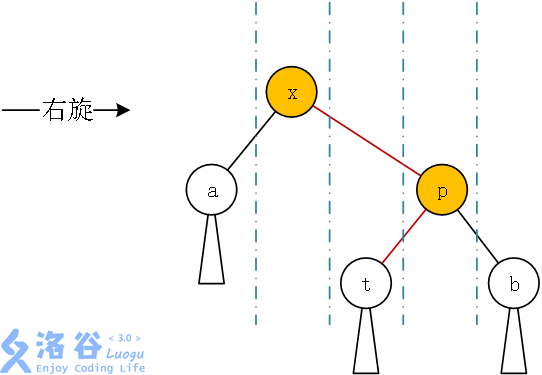2.x与x的父亲与x的爷爷（。。就这么叫好了）三点共线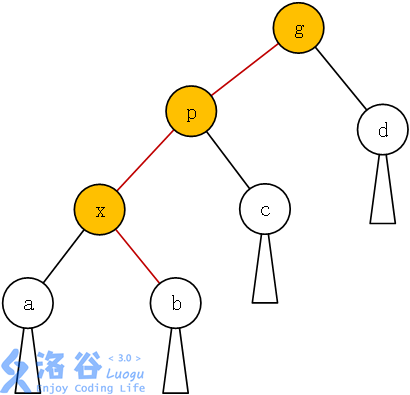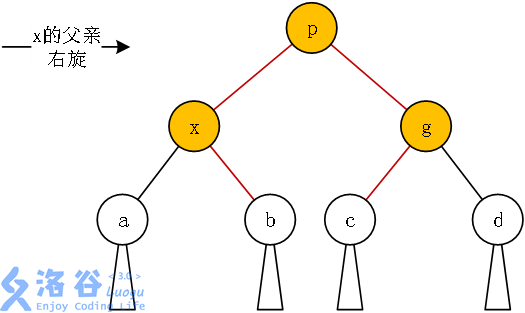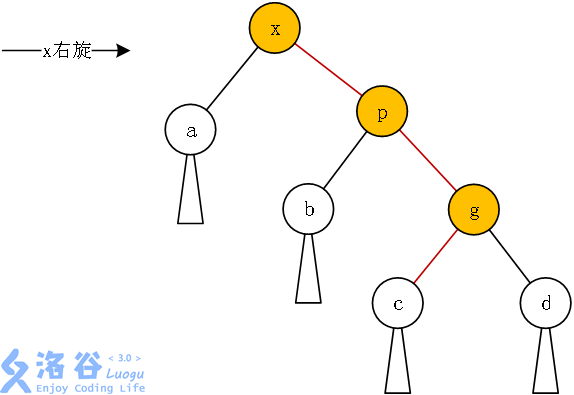3.不共线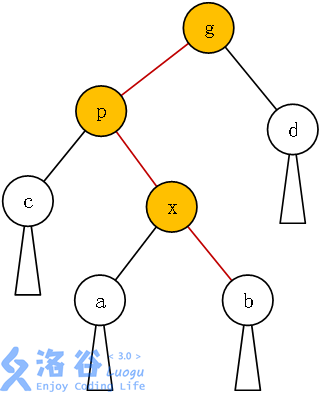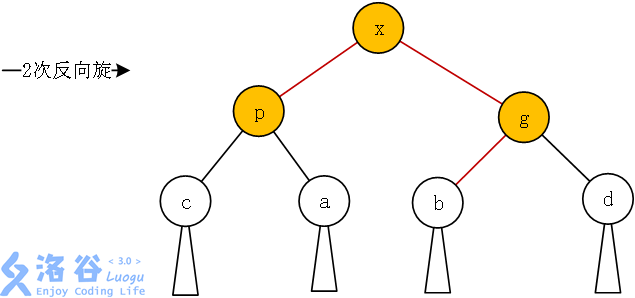int find(int u,int k)
{
if(tr[u].tag) pushdown(u);//区间翻转时交换子树的标记下移
int s=tr[tr[u].s].siz;
if(k==s+1) return u;
if(k<=s) find(tr[u].s,k);
else find(tr[u].s,k-s-1);
}

void pushdown(int x)
{
swap(tr[x].s,tr[x].s);//由于是结构体，所有信息都交换了
tr[tr[x].s].tag^=1;
tr[tr[x].s].tag^=1;
tr[x].tag=0;
}

#include<cstdio>
#include<cstring>
#include<iostream>
#include<algorithm>
using namespace std;
int n,m,rt;
struct node
{
int s;
int val,siz,fa,tag;
void clear()
{
val=0,siz=1;
s=s=0;
tag=0;
}
}tr;
void build(int l,int r,int f)
{
if(l>r) return;
int mid=(l+r)/2;
tr[mid].clear(),tr[mid].val=mid;
if(mid<f) tr[f].s=mid;
else tr[f].s=mid;
tr[mid].fa=f;
if(l==r) return;
build(l,mid-1,mid),build(mid+1,r,mid);
tr[mid].siz+=tr[tr[mid].s].siz+tr[tr[mid].s].siz;
}
void pushdown(int x)
{
swap(tr[x].s,tr[x].s);
tr[tr[x].s].tag^=1;
tr[tr[x].s].tag^=1;
tr[x].tag=0;
}
int find(int u,int k)
{
if(tr[u].tag) pushdown(u);//找的时候同时下传标记
int s=tr[tr[u].s].siz;
if(k==s+1) return u;
if(k<=s) find(tr[u].s,k);
else find(tr[u].s,k-s-1);
}
void rotate(int x,int &k)//旋转，总之就是按上述方法接来接去，想清楚了自然就会打了
{
int y=tr[x].fa,z=tr[y].fa,t;
t=(tr[y].s==x);
if(y==k) k=x;
else tr[z].s[tr[z].s==y]=x;
tr[y].s[t^1]=tr[x].s[t];
tr[tr[y].s[t^1]].fa=y;
tr[x].s[t]=y;tr[y].fa=x;tr[x].fa=z;
tr[y].siz=tr[tr[y].s].siz+tr[tr[y].s].siz+1;
tr[x].siz=tr[tr[x].s].siz+tr[tr[x].s].siz+1;

}
void splay(int x,int &k)//伸展函数，在翻转时目的地节点可能会变，所以用了&
{
while(x!=k)
{
int y=tr[x].fa,z=tr[y].fa;//取父亲和爷爷
if(y!=k)//分三种情况讨论
{
if((x==tr[y].s)^(y==tr[z].s)) rotate(x,k);
else rotate(y,k);
}
rotate(x,k);
}
}
int main()
{
scanf("%d%d",&n,&m);
rt=(n+3)/2,tr.siz=0;
build(1,n+2,rt);
while(m--)
{
int l,r;
scanf("%d%d",&l,&r);
l=find(rt,l),r=find(rt,r+2);
splay(l,rt),splay(r,tr[l].s);
tr[tr[r].s].tag^=1;//记得要给标记
}
for(int i=2;i<=n+1;i++)
printf("%d ",find(rt,i)-1);
return 0;
}
• star
首页